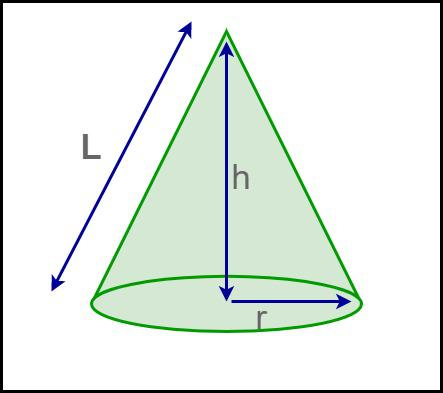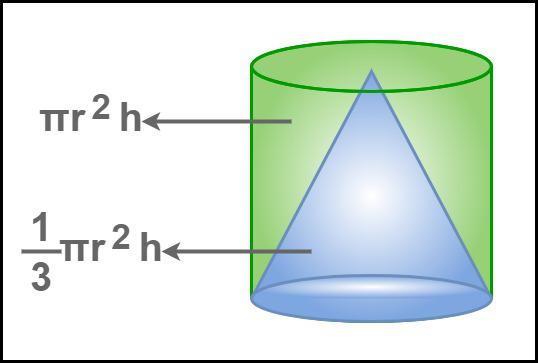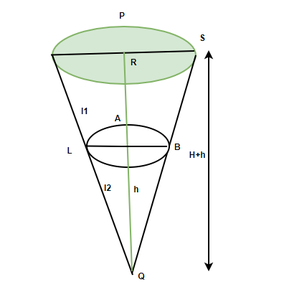Open in App
Not now

# Volume of a Cone

• Last Updated : 14 Sep, 2022

The volume of a cone can be described as the space occupied by the cone or it is the capacity of the cone. Cone is a 3-D object having a circular flat base and a pointed top called an apex or vertex. A cone is considered a figure formed by a non-congruent circular disc that is getting smaller and is a point only at the tip.

A cone is a solid three-dimensional geometric object with a circular base and a sharp edge at the top known as the apex. It has a single face and a single vertex. For a cone, there are no edges. It is a form with a curving top and a round base. A cone has one face, one vertex, and no edges. Its slant height is the length of the line segment from the peak of the cone to any point on the circle of the cone’s base. A right circular cone is one that has its peak directly above the circular base at a perpendicular distance. An oblique cone is one that does not have its apex directly above the circular base. Also, find the surface area for a right circular cone here.

## What is the Volume of a Cone?

A cone’s volume is defined as the amount of space or capacity it fills. The volume of a cone is measured in cubic units such as cm3, m3, in3, and so on. By rotating a triangle around any of its vertices, a cone can be produced. Volume of a cone can also be measured in liters.## Volume of a Cone Formula

A cone is a solid three-dimensional form having a circular base. It’s got a curved surface. The perpendicular height is the distance from the base to the vertex. A cone may be divided into two types: right circular cones and oblique cones. The vertex of the right circular cone is vertically above the center of the base, but the vertex of the oblique cone is not vertically above the center of the base.

The formula for the volume of a cone is given by:

V =where,

r = radius of the cone,

h = height of the cone,

π = 22/7

Also, the relationship between the cone’s volume and slant height by applying Pythagoras’ theorem to it is given by,

h2 + r2 = L2

=> h = √(L2 – r2)

Hence, the volume of cone in terms of its slant height is given by,

V =## Volume of a Cone Derivation

Suppose we have a cone with a circular base whose radius is r and height is h.We know that the volume of a cone is equal to one-third of the volume of a cylinder having the same base radius and height.

So, the volume becomes,

V = 1/3 x Circular Base area x Height

= 1/3 x πr2 x h

=  πr2h/3

This derives the formula for volume of cone.

## How to Find Volume of a Cone?

Let’s consider an example to determine the volume of a cone.

Example: Determine the volume of a cone if the radius of its circular base is 3 cm and the height is 5 cm.

Step 1: Note the radius of the circular base (r) and the height of the cone (h). Here, the radius is 3 cm and the height is 5 cm.

Step 2: Calculate the area of the circular base = πr2. Substitute the value of r and π in the given equation, i.e., 3.14 × (3)2 = 28.26 cm2.

Step 3: We know that the volume of a cone is (1/3) × (area of the circular base) × height of the cone. So, substitute the values in the equation = (1/3) × 28.26 × 5 = 47.1 cm3.

Step 4: Hence, the volume of the given cone is 47.1 cm3.

Using the steps discussed above the volume of a cone can be calculated.

## Volume of Frustum of Cone

The frustum is the sliced part of a cone, the volume of the frustum of the cone is the amount of liquid any frustum can hold. So for calculating the volume, we need to find the difference in the volumes of two cones. For more detail on Frustum of Cone click here.

### Volume of Frustum of Cone Formula

The formula for finding the volume of the frustum of a cone is given by subtracting the volume of two cones. Study the given figure for finding the volume of the frustum.From the above figure, we have, the total height H’ = H+h and the total slant height L =l1 +l2. Radius of the cone = R and radius of the sliced cone = r. Now the volume of the bigger cone = 1/3 π R2 H’ = 1/3 π R2 (H+h)

Volume of the smaller cone = 1/3 πr2h. Volume of the frustum can be calculated by the difference between the two cones, i.e.

Volume of Frustum = 1/3 π R2 H’ -1/3 πr2h
= 1/3π R2 (H+h) -1/3 πr2h
=1/3 π [ R2 (H+h)-r2 h ] ………(1)

Using the properties of similar triangles in Δ QPS and Δ QAB. we have,
R/ r = H+h / h
H+h = (Rh)/r.
Substituting the value of H+h in the formula for the volume of frustum we get,

Volume of frustum = 1/3 π [ R2 (Rh/r)-r2 h ]
= 1/3 π  [R3h/r-r2 h]
= 1/3 π h (R3/r-r2 )
= 1/3 π h (R3-r3 / r)

Volume of Frustum of Cone = 1/3 π h [(R3-r3)/ r]

## Solved Example on Volume of a Cone

Example 1. Find the volume of a cone for a radius of 7 cm and height of 14 cm.

Solution:

We have, r = 7 and h = 14.

Volume of cone = 1/3 πr2h

= (1/3) (22/7) (7) (7) (14)

= (1/3) (7) (7) (2)

= 32.66 cm3

Example 2. Find the volume of a cone for a radius of 5 cm and height of 9 cm.

Solution:

We have, r = 5 and h = 9.

Volume of cone = 1/3 πr2h

= (1/3) (3.14) (5) (5) (9)

= (3.14) (5) (5) (3)

= 235.49 cm3

Example 3. Find the volume of a cone for a radius of 7 cm and height of 12 cm.

Solution:

We have, r = 7 and h = 12.

Volume of cone = 1/3 πr2h

= (1/3) (22/7) (7) (7) (12)

= (22) (7) (4)

= 616 cm3

Example 4. Find the volume of a cone for the radius of 8 cm and height of 15 cm.

Solution:

We have, r = 8 and h = 15.

Volume of cone = 1/3 πr2h

= (1/3) (22/7) (8) (8) (15)

= (1/3) (22/7) (8) (8) (5)

= 335.02 cm3

Example 5. Find the volume of a cone for the diameter of 24 cm and slant height of 13 cm.

Solution:

We have, 2r = 24

=> r = 24/2

=> r = 12

Also, l = 13.

Volume of cone = 1/3 πr2 √(l2 – r2)

= (1/3) (22/7) (12) (12) (√(132 – 122)

= (1/3) (22/7) (12) (12) (5)

= 754.28 cm3

Example 6. Find the volume of a cone for the diameter of 16 cm and slant height of 10 cm.

Solution:

We have, 2r = 16

=> r = 16/2

=> r = 8

Also, l = 10.

Volume of cone = 1/3 πr2 √(l2 – r2)

= (1/3) (22/7) (8) (8) (√(102 – 82)

= (1/3) (22/7) (8) (8) (6)

= 402.048 cm3

Example 7. Find the volume of a cone for the height of 8 cm and the slant height of 17 cm.

Solution:

We have h = 8 and l = 10.

Find the value of r.

r = √(l2 – h2)

= √(172 – 82)

= √(289 – 64)

= 15

Volume of cone = 1/3 πr2h

= (1/3) (22/7) (15) (15) (8)

= (1/3) (22/7) (5) (15) (8)

= 1884.6 cm3

## FAQs on Volume of a Cone

Question 1: What happens to the volume of a cone if its radius and height are doubled?

If r = 2r and h = 2h,

then the volume of a cone is given as:

Volume of a cone = (1/3)π(2r)2(2h) cubic units

V = (⅓)π(4r2)(2h)

V = (8/3)πr2h

Thus, volume of a cone becomes 8 times the original volume i.e. V = (8/3)πr2h,

when its radius and height are doubled.

Question 2: What is the formula for the total surface area of a cone?

Total surface area of a cone is given by the formula πr(l + r) square units, where r is the radius of the circular base and l is the slant height of the cone.

Question 3: What is the formula for the volume of a cone?

Volume of a cone is given by the formula ⅓ πr2 x h cubic units, where r is the radius of the circular base and h is the height of the cone.

Question 4: Find the relation between the Volume of the cylinder and the volume of a cone.

One-third of the volume of a cylinder is equal to the volume of a cone, having the same radius and height.

Question 5: What is the formula for the slant height of a cone?

The slant height of a cone l = √(h2 + r2), where h is the height of the cone and r is the radius of the circular base.

Question 6: What will be the volume of a cone if its radius and height are Halved?

If r = r/2 and h = h/2,

then the volume of a cone is given as:

Volume of a cone = (1/3)π(r/2)2(h/2) cubic units

V = (⅓)π(r2/4)(h/2)

V = (1/24)πr2h

Thus, volume of a cone becomes 1/8 times the original volume i.e. V = (1/24)πr2h,

when its radius and height are halfed.

Question 7: How to find the volume of a cone, if the height and diameter of the cone are given?

Given,

Volume of a cone = (1/3)πr2h cubic units

Where r is radius and h is height

the volume of a cone becomes

V = (1/3)π(d/2)2h cubic units

V = (1/12)πd2h cubic units.

Hence, the formula for the volume of a cone is (1/12)πd2h cubic units, if its height and diameter are given.

### Related Resources

My Personal Notes arrow_drop_up
Related Articles